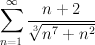# Series converges or diverges

## Homework Statement

Determine whether the series converges or diverges.## The Attempt at a Solution

I got the correct answer but I'm not sure if my method is correct, or if I made so many mistakes that I ended up with the correct answer. If someone could go over this and tell me if my math is perfect or flawed, that would be fantastic.

First I set up a comparison series. The (7/3) comes from taking the n^7 out of the cubed root.

$$\sum_{n=1}^{\infty} \frac{n}{n^\frac{7}{3}} = \sum_{n=1}^{\infty} \frac{1}{n^\frac{4}{3}}$$

This new series is a convergent p-series since p = (4/3), and (4/3) > 1.

So to test if the original series is also convergent, I have to use either the direct comparison test or the limit test. The limit test looks like it would be a pain to do, so I tried the direct comparison test. Unfortunately, the original series is not less than or equal to the new series, so the direct comparison test would not work. So I used the limit test.

$$\lim_{n \to \infty} | \frac{a_{n}}{b_{n}} |$$

$$\lim_{n \to \infty} | \frac{n^{\frac{4}{3}}*n+2}{\sqrt{n^{7}+n^{2}}} |$$

$$\lim_{n \to \infty} | \frac{n^{\frac{7}{3}}+2}{\sqrt{n^{7}+n^{2}}} |$$

Seeing that the highest power up top is equal to the highest power on bottom (7/3), I used to shortcut that the limit will end up being the ratio of those two coefficients (in this case, 1/1 = 1).

So b_n is >0, the limit is 1, which is a positive, finite number, which confirms that the original series is convergent.

I think the radical is throwing me off... I don't know what to do with it. When I try to take the limit do I divide all the terms by n^7, or n^7/3 since it's under the radical? Or is it fine the way I did it?

Last edited:

## Answers and Replies

Pull out n7/3 from the radical, simplify the expression a bit by making the n7/3's cancel, and then take the limit.

Well that's what I'm having difficulty with... pulling terms out of that radical. I've searched the Internet high and low and apparently there is no example of pulling out a term of a radical that is being added.

For example:

$$\sqrt{4^{2}+5^{2}}$$

$$(4^{2}+5^{2})^{1/2}$$

$$4^{2/2}+5^{2/2}$$

$$4^{1}+5^{1}$$

$$4+5$$

$$9$$

And I know that the original definitely does not equal 9, so this can't be a correct method... So I don't really know how I can just pull that n^7 out of that cubed root.

What you reasoned in the original post should be fine. As n grows large, the n^2 term under the radical is insignificant, so the denominator behaves as n^(7/3) and it is clear the ratio a_n / b_n tends to 1. Don't forget to multiply the 2 in the numerator by n^(4/3).

PAllen
Science Advisor
You can also use the comparison test. Just multiply your simple series by e.g. 2. Then for all n greater than some value, the augmented simple series will be larger. This is a common trick you should know.

Ahh, makes sense. Thank you all!

Reply to the algebra question: $\sqrt{4^2 + 5^2}$

$\sqrt{4^2 (1+ \frac{5^2}{4^2})}$

$[4^{2}(1+\frac{5^2}{4^2})]^\frac{1}{2}$

and by the law of exponents

$(a^{m}b^{n})^c = a^{mc}b^{nc}$

$4^{\frac{2}{2}}\sqrt{1+ \frac{5^2}{4^2}}$

Thats it.
OMG I hate writing code, but this is to help others so smile!

Last edited: